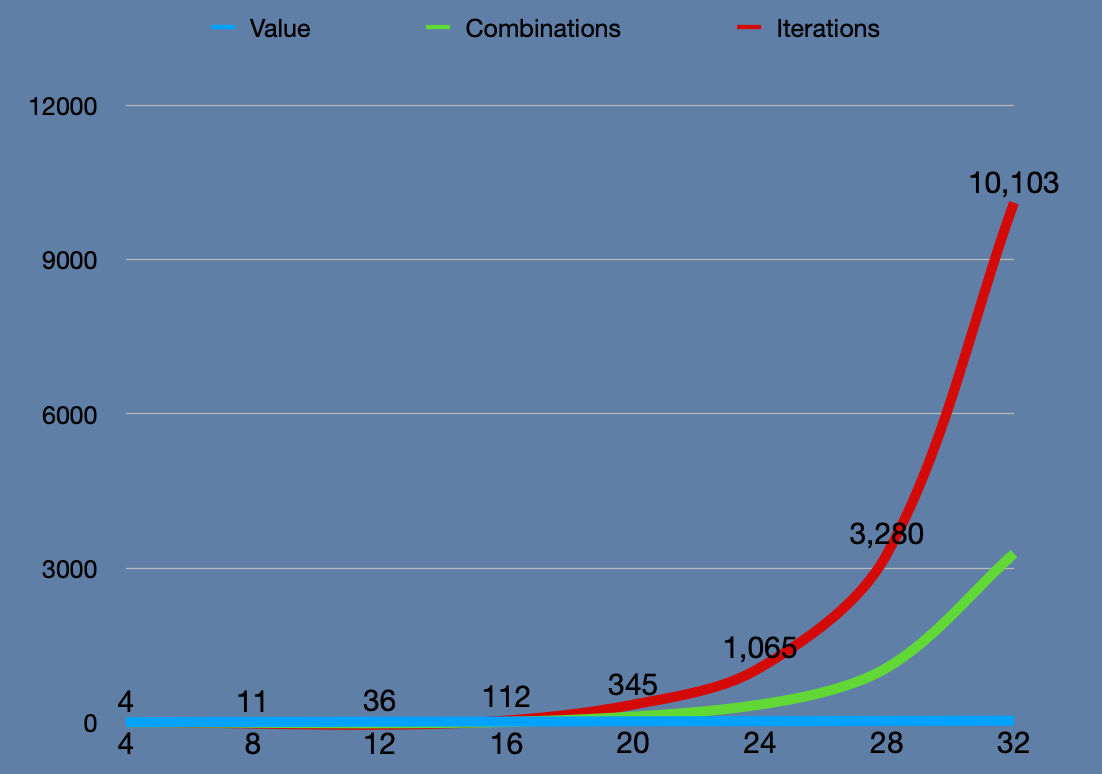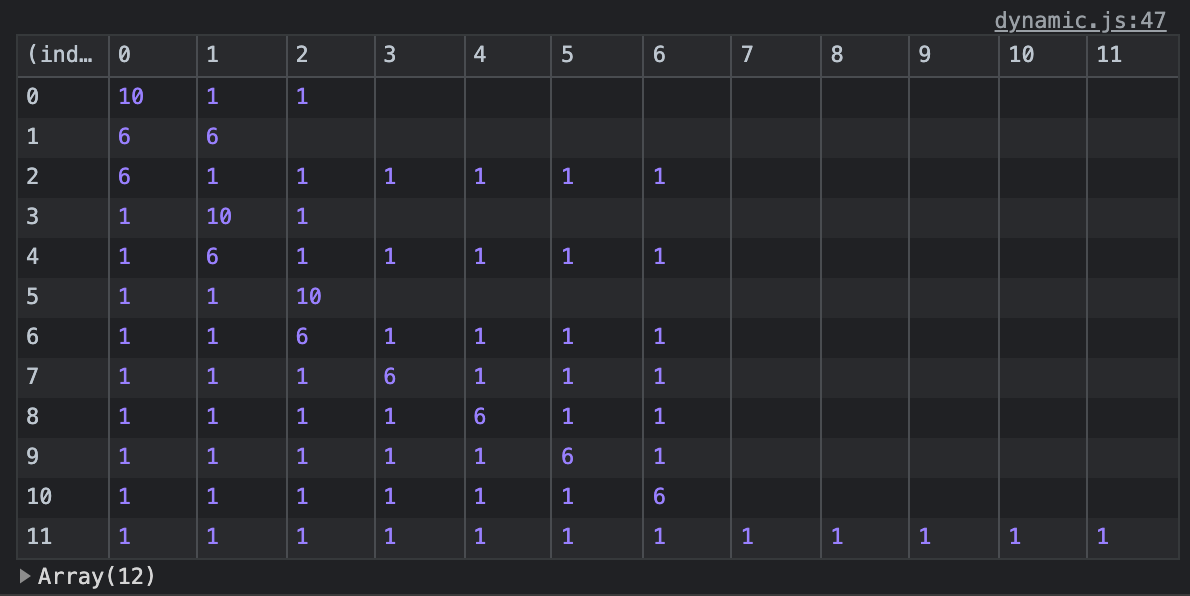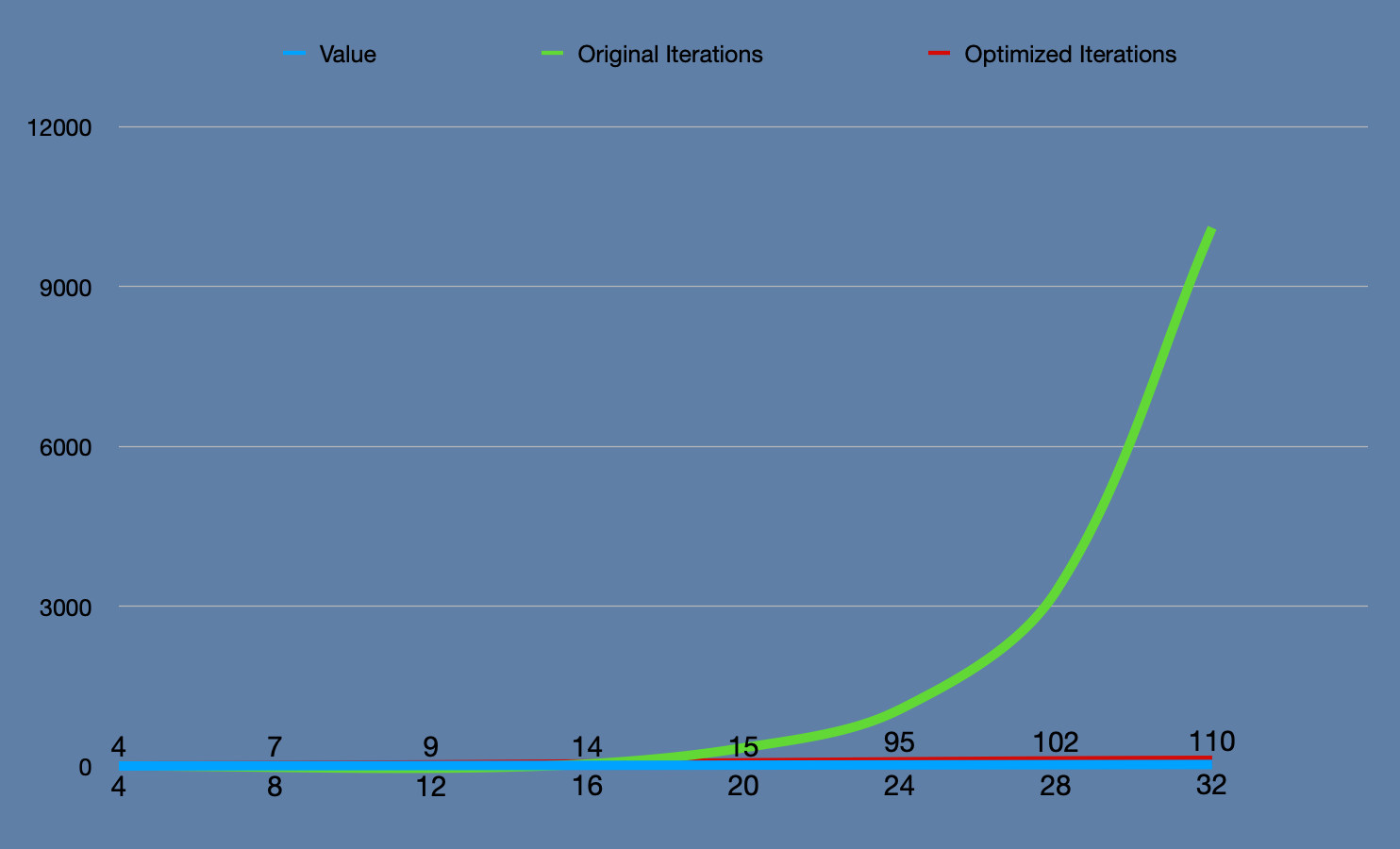# roymosby.me

## Making Change Problem, Part II

Published: Fri, Aug 06, 2021

This is the second part of a 2-part blog post on the coin problem. Click here to go to the first article if you have not read it yet. My goal with this second post is to decrease the computational expense of my original solution.

### The change problem restated

You are given 3 denominations of coins to work with. You are also given a target value. You are supposed to find out the least number of coins that it takes to get to the target value. To keep things simple, it is also assumed that all target values have a solution. You'll always be able to make change.

## Where I left off

I had a brute-force approach that examined all possible combinations of coins. It could then determine the best change combination to make regardless of the coin denominations used.

``const makeChangeClosure = (coins) => {  let combos = []  let shortestCombo = []  let iterations = 0  let cache = {}  	function makeChange(value, aggregator = []) {    iterations++    coins.forEach((coin) => {      let remaining = value - coin;      if(remaining > 0){        makeChange(remaining, [...aggregator, coin])      } else if (remaining === 0) {        combos.push([...aggregator, coin]        if(aggregator.length < shortestCombo.length || shortestCombo.length === 0 ){          shortestCombo = [...aggregator, coin]        }      }    })    return {shortestCombo, combos, iterations, cache}  }	return makeChange}const bruteChange = makeChangeClosure([10,6,1])const bruteChange12 = bruteChange(12)``

## Sample of brute force results

The solution works but it struggles with modestly large values. I crashed by browser when I plugged in 78! This is because it is an exponential - O(n^2) solution.## Examining combinations

I get a sense of how the algorithm proceeds by logging out a sample of combos from a single value. The combos vary in length and trend longer with the smaller the initial coin being worked with.## A closer look into the algorithm

Let's hone in on the workhorse of the algorithm:

``//...if(remaining > 0){	makeChange(remaining, [...aggregator, coin])} else if (remaining === 0) {	combos.push([...aggregator, coin]	if(aggregator.length < shortestCombo.length || shortestCombo.length === 0 ){		shortestCombo = [...aggregator, coin]	}}//...``

This part of `makeChange` sets the base conditions for recursion. If remaining is greater than 0, then it will call `makeChange` again with the remaining value and the updated aggregator. If remaining is 0, then it adds the completed combo to all the other completed combos. Also, if it is shorter than `shortestCombo`, it then updates that value.

The final condition is implicit; if the algorithm overshoots 0 and ends up with a negative value, then that combination is no good. With the way this is written, nothing is done with that bad combo and it just drops off. It kinda feels like dropping litter into the street but I promise you that the browser has a good cleanup crew!

## Time to refactor

Since we are already calculating the shortest combo during recursion, I can adjust the `if/else` statements to take this into account. I need to make sure that it runs at least once since `shortestCombo` starts as an empty array. After the first run, I would compare the length of the `aggregator` to the length of the `shortestCombo` in addition to the current evaluation of `remaining > 0`. Any iteration that has an `aggregator` the same length as the `shortestCombo` can then be abandoned.

2 other important changes:

• I move the iterator to the end of the recursive function
• I ensure the coins are always sorted by decreasing value

``const makeChangeClosure = (coins) => {  //sorts coins to take advantage of the  //tendency for shorter combos to be  //composed of larger coins  coins = coins.sort((a,b) => b-a)  let combos = []  let shortestCombo = []  let iterations = 0  function makeChange(value, aggregator = []){    coins.forEach((coin) => {      let remaining = value - coin;      if (iterations === 0 && remaining > 0){ //new code        makeChange(remaining, [...aggregator, coin]) //new code      } else if (remaining > 0 && aggregator.length < shortestCombo.length){ //added array length evaluation        makeChange(remaining, [...aggregator, coin])      } else if (remaining === 0) {        combos.push([...aggregator, coin].sort((a,b)=> b-a))        if(aggregator.length < shortestCombo.length || shortestCombo.length === 0 ){          shortestCombo = [...aggregator, coin]        }      }    })    iterations++ //moved from top of makeChange    return {shortestCombo, combos, iterations}  }  return makeChange}const bruteChange = makeChangeClosure([10,6,1])const bruteChange12 = bruteChange(12)console.log(`Iterations: \${bruteChange12.iterations}`)console.table(bruteChange12.combos)``

Since I established conditions where not every single combo needs evaluated, I cut out a lot of the algorithm's unneeded work. It is still a brute force approach but it's smarter.

value brute-force iterations optimized algorithm's iterations
12 36 9

### Sample Comparison old vs optimized

The difference is so remarkable that it barely registers when charted along with the original solution. It still balloons faster than the input value but not nearly so much.

value original iterations optimized iterations
4 4 4
8 11 7
12 36 9
16 112 14
20 345 15
24 1065 95
28 3280 102
32 10103 110This time around, I am not afraid to enter 78 as a value! It's hefty at 29385 iterations to come up with a winning combo but does not crash my browser like it initially did.